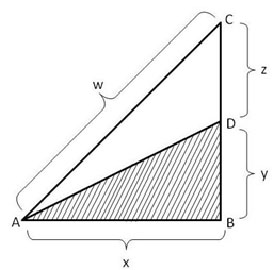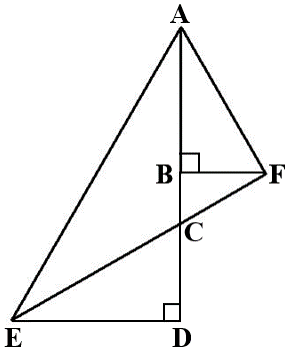# Geometry Practice Questions

Angles, Triangles, Quadrilaterals, Circles, Semi circles and squares. A collection of curated practice questions on Geometry that typically appear in TANCET, GMAT, GRE, CAT and other B School admission tests. Detailed explanatory answers and shortcuts, wherever applicable, are provided for each of the questions.

1. What is the ratio measures of the in-radius, circum-radius and one of the ex-radius of an equilateral triangle?

1. 1 : 2 : 5
2. 1 : 3 : 5
3. 1 : 2 : 3
4. 1 : 1.4142 : 2
2. If in a triangle ABC, $$frac{cos A}{a}$ = $\frac{cos B}{b}$ = $\frac{cos C}{c}$, then what can be said about the triangle? 1. Right angled triangle 2. Isosceles triangle 3. Equilateral triangle 4. Nothing can be inferred 3. If the largest angle in a triangle is 70o, what is least possible value of the smallest angle of the triangle? 4. If the shaded area is one half the area of triangle ABC and angle ABC is right angle, then the length of line segment AD is1. $\frac{1}{2}$w 2. c 3. $\sqrt {{2x}^{2}+{z}^{2}}$ 4. $\sqrt {{w}^{2}-{3y}^{2}}$ 5. $\sqrt {{y}^{2}+{z}^{2}}$ 5. Which of the following is incorrect? 1. An incentre is a point where the angle bisectors meet. 2. The median of any side of a triangle bisects the side at right angle. 3. The point at which the three altitudes of a triangle meet is the orthocentre 4. The point at which the three perpendicular bisectors meet is the centre of the circumcircle. 6. A circle of maximum possible size is cut from a square sheet. Subsequently, a square of maximum possible size is cut from the resultant circle. What will be area of the final square? 1. 75% of the of the original square 2. 50% of the size of the original square 3. 75% of the size of the circle 4. 25% of the size of the original square 7. What is the area of the largest triangle that can be fitted into a rectangle of length 'l' units and width 'w' units? 1. $\frac{lw}{3}$ 2. $\frac{2lw}{3}$ 3. $\frac{3lw}{4}$ 4. $\frac{lw}{2}$ 8. Each interior angle of a regular polygon is 120 degrees greater than each exterior angle. How many sides are there in the polygon? 9. A stairway 10ft high is such that each step accounts for half a foot upward and one-foot forward. What distance will an ant travel if it starts from ground level to reach the top of the stairway? 1. 30 ft 2. 33 ft 3. 10 ft 4. 29 ft 10. Find the area of the sector covered by the hour hand after it has moved through 3 hours if the length of the hour hand is 7cm. 1. 77 sq.cm 2. 38.5 sq.cm 3. 35 sq.cm 4. 70 sq.cm 11. Find the number of triangles in an octagon. 1. 326 2. 120 3. 56 4. Cannot be determined 12. What is the circum radius of a triangle whose sides are 7, 24 and 25 respectively? 13. What is the measure of in radius of the triangle whose sides are 24, 7 and 25? 1. 12.5 2. 3 3. 6 4. None of these 14. If ABC is a right angle triangle with angle A = 900 and 2s = a + b + c, where a > b > c where notations have their usual meanings, then which one of the following is correct? 1.$s – b) (s – c) > s (s – a)
2. (s – a) (s – c) > s (s – b)
3. (s – a) (s – b) < s (s – c)
4. 4s (s – a) (s – b) (s – c) = bc
15. If the sum of the interior angles of a regular polygon measures up to 1440 degrees, how many sides does the polygon have?

1. 10 sides
2. 8 sides
3. 12 sides
4. 9 sides
16. What is the measure of the circum radius of a triangle whose sides are 9, 40 and 41?

17. In the diagram below, CD = BF = 10 units and ∠CED = ∠BAF = 30o. What would be the area of triangle AED? (Note: Diagram below may not be drawn to scale.)1. 100 x ($$sqrt2$ + 3) 2. $\frac {100} {\left$ {{$sqrt {3+4}}} \right$}) 3. $\frac {50} {\left$ {{$sqrt {3+4}}} \right$}) 4. 50 x$$\sqrt3$ + 4)

## Online TANCET MBA CourseTry it Free!

Register in 2 easy steps and start learning in 5 minutes!